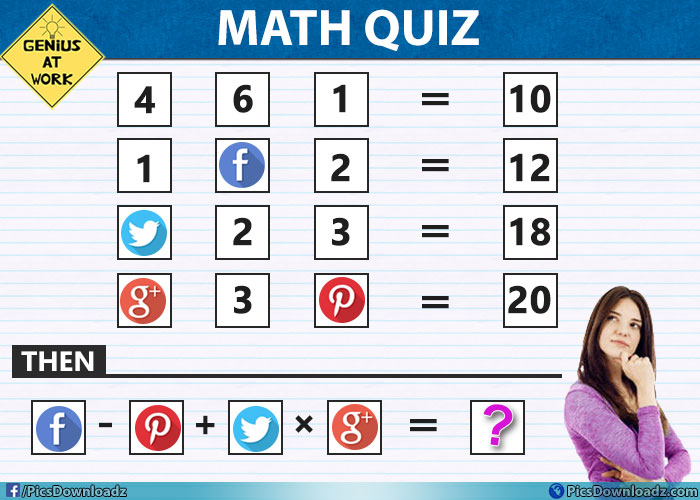This post may contain affiliate links. This means if you click on the link and purchase the item, We will receive an affiliate commission at no extra cost to you. See Our Affiliate Policy for more info.

# Facebook – Pin + Twitter × Google = ?? Solve this Viral Social Maths Puzzle Image

0
2976

### Solve the puzzle if you are a genius! 95% will fail to answer this interesting social maths puzzle! Only for geniuses maths puzzle picture!

This is one of the best puzzles in our list. You will have to find out the values of these social icons and then put their values in the last equation and find which number replace the question mark.

Pretty simple and a little bit confusing. If you already solved the puzzles like this one here, which is a little bit similar to this puzzle, then you will understand how to solve the first part of the puzzle. Best of luck.

Puzzle Question:

⇒ 4,  6,  1 = 10

⇒ 1,   F,  2 = 12

⇒ T , 2,  3 = 18

⇒ G,  3,  P = 20

2. Which number replace the question mark:

⇒ F – P + T × G = ??

## Maths Puzzles Image:

[mashshare]Solved this problem? Let us know how active your brain is, share your answer via the comment section below. Need some hint or options? Check these options.

Just for fun 😉

Options level:

1. 04
2. 03
3. 09
4. 10
5. none of above
6. I don’t know
7. This puzzle makes no sense

Keep scrolling for the solution of this puzzle.

A

N

S

W

E

R

.

H

E

R

E

.

Oh, so you choose option 8. what? just want to confirm! Ok whatever!

### 1st Part of the Puzzle:

So, here is our question:

1⇒  4, 6, 1 = 10

2⇒  1, F, 2 = 12

3⇒  T, 2, 3 = 18

4⇒  G, 3, P = 20

The logic this puzzle problem following is:

⇒  4, 6, 1 = 10    ⇒    (4 + 6) × 1 = 10 (Apply the same logic on every equations)

⇒  1, F, 2 = 12    ⇒    (1 + F) × 2 = 12    ⇒    (1 + 5) × 2 = 12.    Facebook (F) = 5

⇒  T, 2, 3 = 18   ⇒    (T + 2) × 3 = 18   ⇒    (4 + 2) × 3 = 18.    Twitter (T) = 4

⇒  G, 3, P = 20  ⇒    (G + 3) × P = 20  ⇒

In this equation, if we assume G = 1 and P = 5,  or G = 2 and P = 4, in both case we got the same result i.e 20. But there is a trick to find the correct value of G and P.

As we can see the last digit of the each equation in Left Hand Side (LHS) is following the sequence of 1,2,3,.. and so on. So our next digit will be 4 which equals to P and now we can find the value of G.

(G + 3) × 4 = 20  ⇒  (2 + 3) × 4 = 20.   So the correct value of Google (G) = 2 and Pinterest (P) = 4

### 2nd Part of the Puzzle:

Which number replace the question mark?

⇒ F – P + T × G = ??  (As we know now; F = 5, T = 4, G = 2 and P = 4)

⇒ 5 – 4 + 4 × 2  ⇒   5 – 4 + (4 × 2)  (Hope you know BODMAS rule) ⇒   5 – 4 + 8 = 1 + 8 = 9 Answer.

If you have more solution, let us know through comments.

Found this one is interesting? don’t forget to pin or share this puzzle image with your friends. Like us on facebook for more awesome puzzles and funny stories.

If you love these kinds of tricky and confusing puzzles and riddles, you should definitely join our facebook group “Only for Genius”: